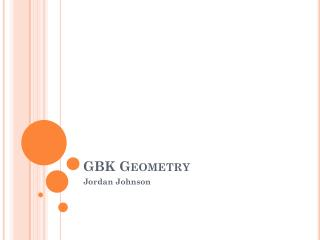DownloadDownload PresentationGBK Geometry

# GBK Geometry

Download Presentation## GBK Geometry

- - - - - - - - - - - - - - - - - - - - - - - - - - - E N D - - - - - - - - - - - - - - - - - - - - - - - - - - -
##### Presentation Transcript

1. GBK Geometry Jordan Johnson

2. Today’s plan • Greeting • Review Asg #85: • From Ch. 13, Lesson 1 (pp. 530-535): • Exercises 1-14, 46-48. • From Ch. 13, Lesson 2 (pp. 537-539): • Easy: 1-2, 6-12. Med: 29-31. • Bonus: Set III from Ch. 13, Lesson 1. • Lesson: Regular Polygons – Conclusion • Homework / Questions • Clean-up

3. Regular Polygon Perimeter & Area

4. Regular Polygon Perimeter & Area

5. Regular Polygon Perimeter & Area

6. Regular Polygon Perimeter & Area or 6r (or we could write r⁄2 instead) or 3⁄2 or (33⁄2)r2

7. Regular Polygon Perimeter & Area

9. A 10,000-gon • n = 10,000 ☺ • Central radius/apothem angle = 180°⁄10,000 • Side length = 2r sin(180°⁄10,000) • Apothem = r cos(180°⁄10,000) • Perimeter = 20,000r sin(180°⁄10,000) • Area = 10,000r2 sin(180°⁄10,000)cos(180°⁄10,000)

11. 10,000-gon n-gon • Central radius/apothem angle = 180°⁄10000 = 180°⁄n • Side length = 2r sin(180°⁄10000) = 2r sin(180°⁄n) • Apothem = r cos(180°⁄10000) = r cos(180°⁄n) • Perimeter = 20,000r sin(180°⁄10000) = 2nr sin(180°⁄n) • Area = 10000r2 sin(180°⁄10000)cos(180°⁄10000) = nr2 sin(180°⁄n)cos(180°⁄n)

12. Perimeter = 2nr sin(180°⁄n) Area = nr2 sin(180°⁄n)cos(180°⁄n) What happens to all thisas n gets reallybig?

13. Perimeter = 2nr sin(180°⁄n) Area = nr2 sin(180°⁄n)cos(180°⁄n) What happens to all thisas n gets reallybig? Let’s isolate the parts with n…

14. Perimeter = 2nr sin(180°⁄n) = 2(n sin(180°⁄n))r Area = nr2 sin(180°⁄n) cos(180°⁄n) = (n sin(180°⁄n) cos(180°⁄n))r2

15. Perimeter = 2nr sin(180°⁄n) = 2(n sin(180°⁄n))r Area = nr2 sin(180°⁄n) cos(180°⁄n) = (n sin(180°⁄n) cos(180°⁄n))r2

16. Calculator time…

17. Calculator time…

18. Calculator time…

19. As N gets large… • n sin(180°⁄n) approaches… • n sin(180°⁄n) cos(180°⁄n) approaches… 

20. Perimeter = 2(n sin(180°⁄n))r  2r Area = (n sin(180°⁄n) cos(180°⁄n))r2  r2

21. Full Circle For a given radius r… The area of a circle is the limit thatthe area of an n-sided polygonapproaches as n grows. The circumference is the limit thatthe perimeter of an n-sided polygon approaches as n grows.

22. Homework • Final review – due the day of the final. • Make a note card for the final. • Journal Entry #16: • What is one helpful thing you learned in geometry class about math this year? • What is one helpful thing you learned in geometry class that wasn't about math this year? • If you could change one thing about the class, what would it be? • If you could have done one thing differently, what would it be? • What advice would you give to another student who is going to take this class? • Due the end of finals week.

23. Clean-up / Reminders • Pick up all trash / items. • Push in chairs (at front and back tables). • See you tomorrow!